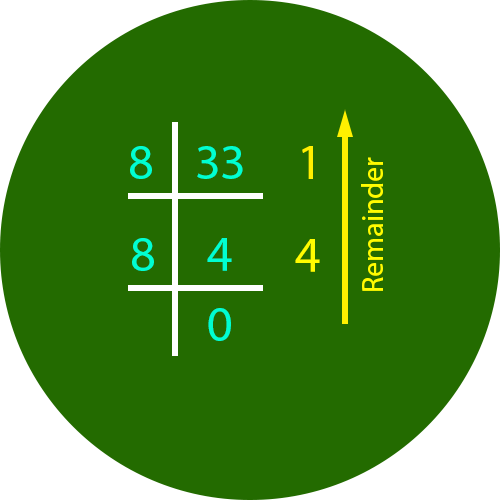﻿ Decimal to Octal Converter | Decimal to Octal Calculator

# Decimal to Octal Converter

Use our Decimal to Octal converter a free online calculator / conversion tool to convert decimal value into octal number system.

#### Decimal Number System

Decimal number system has only ten (10) digits from 0 to 9. Every number (value) represents with 0,1,2,3,4,5,6, 7,8 and 9 in this number system. The base of decimal number system is 10, because it has only 10 digits

#### Octal Number System

Octal number system has only eight (8) digits from 0 to 7. Every number (value) represents with 0,1,2,3,4,5,6 and 7 in this number system. The base of octal number system is 8, because it has only 8 digits.

### Decimal to Octal Conversion

#### How to Convert Decimal to Octal Values

The best method used in decial to octal conversion is the process of successive division. Divide the decimal number by 8; the remainder is the LSB of the octal number. If the quotation is zero, the conversion is complete. Otherwise repeat step (a) using the quotation as the decimal number. The new remainder is the next most significant bit of the octal number.

Example : Convert the decimal number 9410 into its octal equivalent.

First divide 94 by 8; the result is 11 as quotation with 6 as remainder.

Now make last quotation 11 as the new decimal number and divide 11 by 8; the result is 1 as quotation with 3 as remainder.

Now make last quotation 1 as the new decimal number and divide 1 by 8; the result is 0 as quotation with 1 as remainder.

Starting from the last remainder whose quotation is zero, treat it as the MSB and write all remainders in reverse sequence after that we get the answer; 136

So, 9410 = 1368

### Standard number system conversion table

0 0000 0 0
1 0001 1 1
2 0010 2 2
3 0011 3 3
4 0100 4 4
5 0101 5 5
6 0110 6 6
7 0111 7 7
8 1000 10 8
9 1001 11 9
10 1010 12 A
11 1011 13 B
12 1100 14 C
13 1101 15 D
14 1110 16 E
15 1111 17 F### Spreading Knowledge Across the World

USA - United States of America  Canada  United Kingdom  Australia  New Zealand  South America  Brazil  Portugal  Netherland  South Africa  Ethiopia  Zambia  Singapore  Malaysia  India  China  UAE - Saudi Arabia  Qatar  Oman  Kuwait  Bahrain  Dubai  Israil  England  Scotland  Norway  Ireland  Denmark  France  Spain  Poland  and  many more....